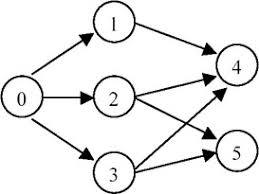# 1145. Data Structure - Topological SortingTopological Sorting

Topological Sorting and related questions.

## 1. Topological Sorting

In computer science, a topological sort or topological ordering of a directed graph is a linear ordering of its vertices such that for every directed edge uv from vertex u to vertex v, u comes before v in the ordering.

• indegree
• outdegree

### 1.1 Problem Description

Given an directed graph, a topological order of the graph nodes is defined as follow:

• For each directed edge A -> B in graph, A must before B in the order list.
• The first node in the order can be any node in the graph with no nodes direct to it.

Find any topological order for the given graph.

Example: For graph as follow:The topological order can be:

[0, 1, 2, 3, 4, 5]
[0, 2, 3, 1, 5, 4]
...


### 1.2 Solution

Calculate InDegree, use BFS approach.

public class DirectedGraphNode {
int label;
List<DirectedGraphNode> neighbors;
public DirectedGraphNode(int x) {
label = x;
neighbors = new ArrayList<>();
}
}

public class TopologicalSorting {
/*
* @param graph: A list of Directed graph node
* @return: Any topological order for the given graph.
*/
public List<DirectedGraphNode> topSort(List<DirectedGraphNode> graph) {
if (graph == null || graph.size() == 0) {
return null;
}
// calculate indegree
Map<DirectedGraphNode, Integer> map = new HashMap<>();
for (DirectedGraphNode node : graph) {
for (DirectedGraphNode neighbor : node.neighbors) {
map.put(neighbor, map.getOrDefault(neighbor, 0) + 1);
}
}

List<DirectedGraphNode> ans = new ArrayList<>();
// queue
for (DirectedGraphNode node : graph) {
if (!map.containsKey(node)) {
queue.offer(node);
}
}

// bfs
while (!queue.isEmpty()) {
DirectedGraphNode node = queue.poll();
for (DirectedGraphNode neighbor : node.neighbors) {
map.put(neighbor, map.get(neighbor) - 1);
if (map.get(neighbor) == 0) {
queue.offer(neighbor);
}
}
}

return ans;
}
}


## 2. Course Schedule

### 2.1 Problem Description

There are a total of numCourses courses you have to take, labeled from 0 to numCourses-1.

Some courses may have prerequisites, for example to take course 0 you have to first take course 1, which is expressed as a pair: [0,1].

Given the total number of courses and a list of prerequisite pairs, is it possible for you to finish all courses?

Example 1:

Input: numCourses = 2, prerequisites = [[1,0]]
Output: true
Explanation: There are a total of 2 courses to take.
To take course 1 you should have finished course 0. So it is possible.


Example 2:

Input: numCourses = 2, prerequisites = [[1,0],[0,1]]
Output: false
Explanation: There are a total of 2 courses to take.
To take course 1 you should have finished course 0, and to take course 0 you should
also have finished course 1. So it is impossible.


### 2.2 Solution

Topological Sorting, check if the number of visited course during BFS are same with the total number of courses.

public boolean canFinish(int numCourses, int[][] prerequisites) {
if (numCourses <= 0) {
return false;
}
if (prerequisites == null || prerequisites.length == 0) {
return true;
}

Map<Integer, List<Integer>> graph = new HashMap<>();

int[] indegree = new int[numCourses];
for (int[] pre : prerequisites) {
indegree[pre]++;
if (!graph.containsKey(pre)) {
graph.put(pre, new ArrayList<>());
}
}

for (int i = 0; i < numCourses; i++) {
if (indegree[i] == 0) {
queue.offer(i);
}
}

int count = 0;
while (!queue.isEmpty()) {
int course = queue.poll();
count++;
if (graph.containsKey(course)) {
for (Integer nextCourse : graph.get(course)) {
indegree[nextCourse]--;
if (indegree[nextCourse] == 0) {
queue.offer(nextCourse);
}
}
}
}

return count == numCourses;
}


## 3. Alien Dictionary

### 3.1 Problem Description

There is a new alien language which uses the latin alphabet. However, the order among letters are unknown to you. You receive a list of non-empty words from the dictionary, where words are sorted lexicographically by the rules of this new language. Derive the order of letters in this language.

• You may assume all letters are in lowercase.
• The dictionary is invalid, if a is prefix of b and b is appear before a.
• If the order is invalid, return an empty string.
• There may be multiple valid order of letters, return the smallest in normal lexicographical order

Examples:

Example 1:

Input：["wrt","wrf","er","ett","rftt"]
Output："wertf"
Explanation：
from "wrt"and"wrf" ,we can get 't'<'f'
from "wrt"and"er" ,we can get 'w'<'e'
from "er"and"ett" ,we can get 'r'<'t'
from "ett"and"rftt" ,we can get 'e'<'r'
So return "wertf"


Example 2:

Input：["z","x"]
Output："zx"
Explanation：
from "z" and "x"，we can get 'z' < 'x'
So return "zx"


### 3.2 Solution

Compare each word with its neighbor to calculate the indegree of each appeared character, then use topological sorting to find the letters in sequence.

/**
* @param words: a list of words
* @return: a string which is correct order
*/
public String alienOrder(String[] words) {
if (words == null || words.length == 0) {
return "";
}

// initialize degree
Map<Character, Integer> indegree = new HashMap<>();
for (String word : words) {
for (char c : word.toCharArray()) {
indegree.put(c, 0);
}
}

Map<Character, List<Character>> graph = new HashMap<>();
for (int i = 1; i < words.length; i++) {
String word1 = words[i - 1];
String word2 = words[i];
for (int j = 0; j < Math.min(word1.length(), word2.length()); j++) {
char c1 = word1.charAt(j);
char c2 = word2.charAt(j);
if (c1 != c2) {
if (!graph.containsKey(c1)) {
graph.put(c1, new ArrayList<>());
}
indegree.put(c2, indegree.get(c2) + 1);
break;
}
}
}

Queue<Character> queue = new PriorityQueue<>();
for (char c : indegree.keySet()) {
if (indegree.get(c) == 0) {
queue.offer(c);
}
}

StringBuilder sb = new StringBuilder();
while (!queue.isEmpty()) {
char c = queue.poll();
sb.append(c);
if (graph.containsKey(c)) {
for (char nextchar : graph.get(c)) {
indegree.put(nextchar, indegree.get(nextchar) - 1);
if (indegree.get(nextchar) == 0) {
queue.offer(nextchar);
}
}
}
}

return sb.length() == indegree.size() ? sb.toString() : "";
}